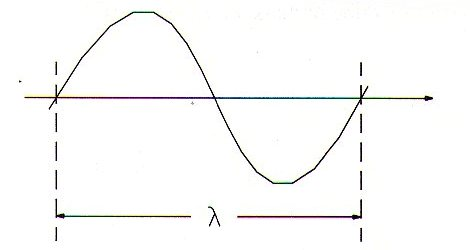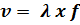### DatasheetsN° of component

## Sound Wavelength (MA198E)

The velocity of sound waves advances is called wave velocity. The velocity depends on the properties of the medium and in some cases on the frequency. The dependence of the wave velocity on the frequency is called dispersion of the velocity.

The wwavelength is defined as the distance travelled by the wave in one period. In consequence, the wavelength depends on the velocity of the wave on the considered medium.FormulaWhere:

λ is the wavelength in meters (m)

v is the propagation velocity in meters per second (m/s)

f is the frequency in hertz (Hz)